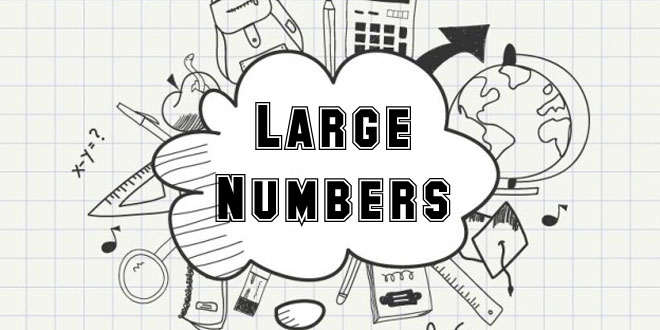Tuesday , May 26 2020# NCERT 5th Class (CBSE) Mathematics: Large Numbers

## Large Numbers

We use only 10 digits in our place value system to write any number, no matter how large it is. The value of the digit depends on its place in the number.

Can you read the number in the following sentence?

India is the seventh largest country in the world with an area of 32, 87, 263 sq. km.The number given above can written in different ways.

Word from: Thirty-two lakh, eighty-seven thousand, two hundred sixty-three.

Long expanded from: (3×10,oo,ooo)+(2×1,oo,ooo)+(8×10,000)+(7×,1000)+(2×100)+(6×1o)+(3×1)

Short expanded from: 30,00,000+2,oo,ooo+80,000+7,000+200+60+3

Standard from: 32,87,263

#### Question: Write the numbers in word from, long expanded from and short expanded from.

(a) 2038987  (b) 198006  (c) 30706  (d) 8609645  (e) 9900051

Answer: (a) Word from: Twenty lakh, thirty-eight thousand, nine hundred eighty-seven

Long expanded from: (2×10,00,000)+(3×10,ooo)+(8×1ooo)+(9×1oo)+(8×10)+(7×1)

Short expanded from: 20.00,000+30,000+8000+900+80+7

(b)  Word from: One lakh, ninety-eight thousand, six

Long expanded from: (1×1,oo,000)+(9×10,000)+(8×1ooo)+(6×1)

Short expanded from: 1,oo,ooo+90,ooo+8000+6

(c) Word from: Thirty thousand, seven hundred six.

Long expanded from: (3×10,000)+(7×100)+(6×1)

Short expanded from: 30,000+700+6

(d) Word from: Eighty-six lakh, nine thousand, six hundred forty-five

Long expanded from: (8×1o,oo,ooo)+(9×10,000)+(6×100)+(4×10)+(5×1)

Short expanded from: 8o,oo,ooo+60,000+40+5

(e) Word from: Ninety-nine lakh, fifty-one

Long expanded from: (9×1o,00,000)+(9×1,00,000)+(50×1)+(1×1)

Short expanded from: 99,00,000+50+1

## 10th Class CBSE Mathematics 2018-19

10th Class CBSE Mathematics 2018-19 Time: 3 hours M.M.: 80 Class: 10th Subject: Mathematics Date: 07/03/2019 …

1.2.